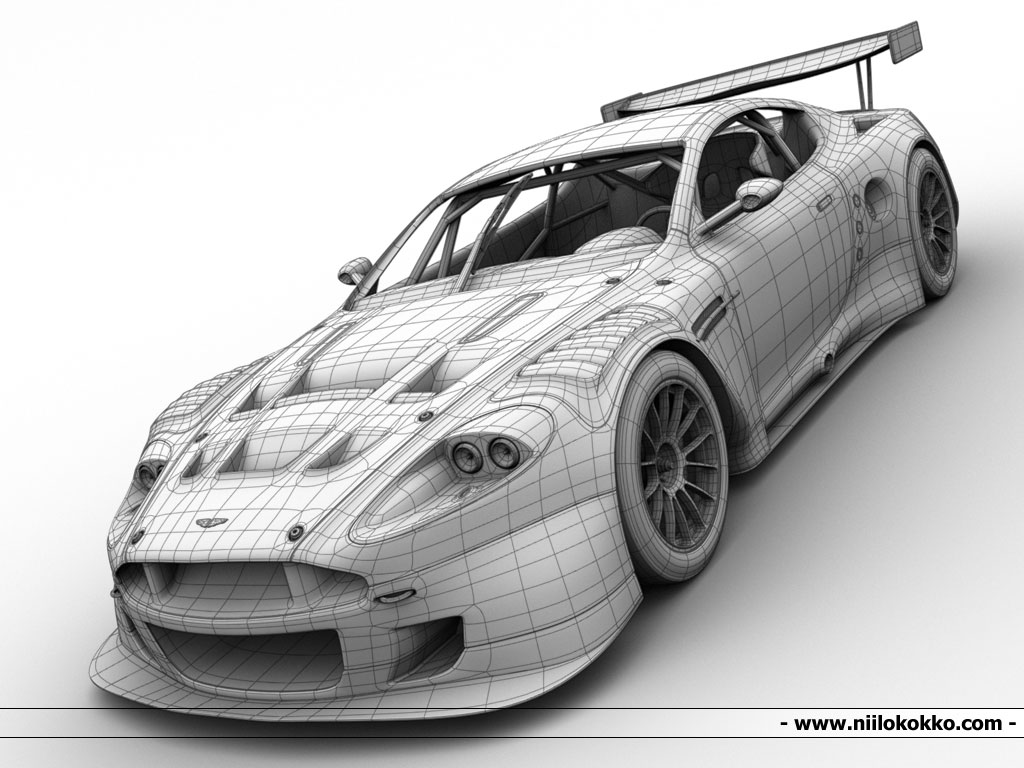# The four areas

 In order to make this huge world more approachable we are going to break it into 4 main areas of experimentation. 1. Character designYou can read a brief introduction here. And see some examples here. 2. Motion graphicsLearn a bit more of what it means here. And see some cool graphics here. 3. AnimationAn introduction here.  You can see some examples of animations here. 4. 3D Modelling (with the possibility of 3D printing)An introduction here. You can see some examples of cool models here. Image source.